### Home > CALC > Chapter Ch10 > Lesson 10.1.6 > Problem10-54

10-54.
1. Examine the integrals below. Consider the multiple tools available for integrating and use the best strategy. After evaluating each integral, write a short description of your method. Homework Help ✎

1.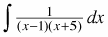2.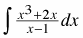3.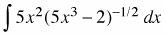4.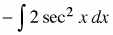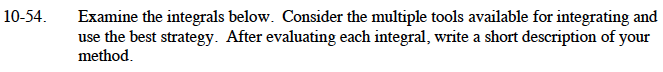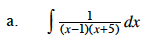Use partial fraction decomposition.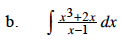Rewrite the integrand by using polynomial division.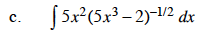Use substitution. Let u = 5x3 – 2.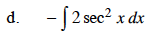$\frac{d}{dx}\tan(x)=\sec^2(x)$